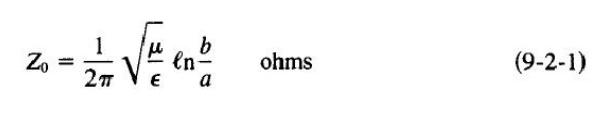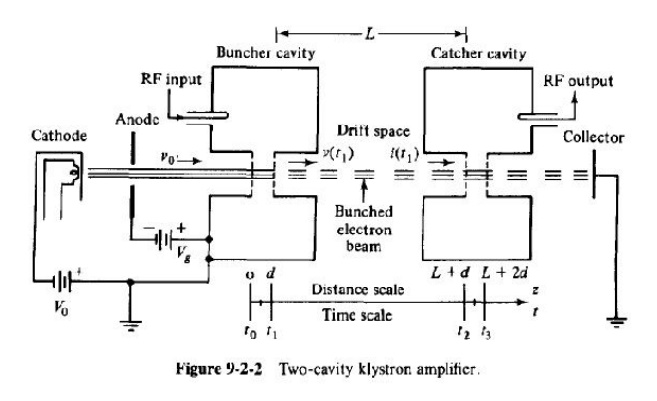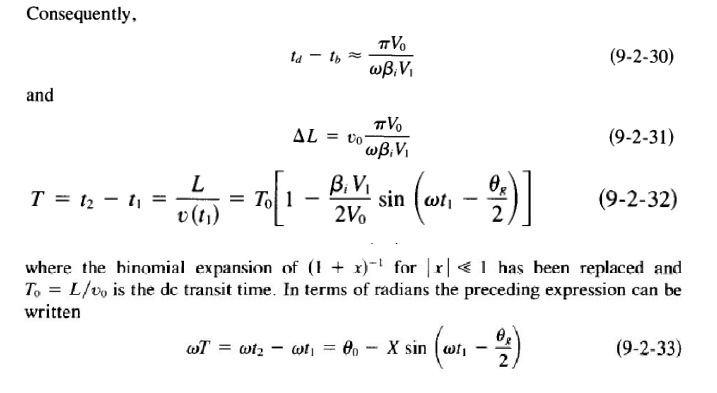Home | | RF and Microwave Engineering | Operation of Multicavity Klystron

# Operation of Multicavity Klystron

Two cavity klystron: The two-cavity klystron is a widely used microwave amplifier operated by the principles of velocity and current modulation.

OPERATION OF MULTICAVITY KLYSTRON

Two cavity klystron:

The two-cavity klystron is a widely used microwave amplifier operated by the principles of velocity and current modulation. All electrons injected from the cathode arrive at the first cavity with uniform velocity. Those electrons passing the first cavity gap at zeros of the gap voltage (or signal voltage) pass through with unchanged velocity; those passing through the positive half cycles of the gap voltage undergo an increase in velocity; those passing through the negative swings of the gap voltage undergo a decrease in velocity.

As a result of these actions, the electrons gradually bunch together as they travel down the drift space. The variation in electron velocity in the drift space is known as velocity modulation. The density of the electrons in the second cavity gap varies cyclically with time.

The electron beam contains an ac component and is said to be current-modulated. The maximum bunching should occur approximately midway between the second cavity grids during its retarding phase; thus the kinetic energy is transferred from the electrons to the field of the second cavity.

The electrons then emerge from the second cavity with reduced velocity and finally terminate at the collector. The charateristics of a two-cavity klystron amplifier are as follows:

2.Power output: average power ( CW power) is up to 500 kW and pulsed power is up to 30 MW at 10 GHz.

Reentrant Cavities

The coaxial cavity is similar to a coaxial line shorted at two ends and joined at the center by a capacitor. The input impedance to each shorted coaxial line is given bywhere e is the length of the coaxial line.

Substitution of Eq. (9-2-l) in (9-2-2) results inThe inductance of the cavity is given b

At resonance the inductive reactance of the two shorted coaxial lines in series is equal in magnitude to the capacitive reactance of the gap. That is, wL = 1/(wCg).

Thus where v = 1/yr;;; is the phase velocity in any medium

Velocity-Modulation Process

When electrons are first accelerated by the high de voltage Vo before entering the buncher grids, their velocity is uniform:In Eq. (9-2-10) it is assumed that electrons leave the cathode with zero velocity. When a microwave signal is applied to the input terminal, the gap voltage between the buncher grids appears aswhere V1 is the amplitude of the signal and V1 << Vo is assumed.

In order to find the modulated velocity in the buncher cavity in terms of either the entering time to or the exiting time t1 and the gap transit angle 88 as shown in Fig. 9-2-2 it is necessary to determine the average microwave voltage in the buncher gap as indicated in Fig. 9-2-6. Since V1 << Vo , the average transit time through the buncher gap distance d isIt can be seen that increasing the gap transit angle 0g decreases the coupling between the electron beam and the buncher cavity; that is, the velocity modulation of the beam for a given microwave signal is decreased. Immediately after velocity modulation, the exit velocity from the buncher gap is given byBunching Process

Once the electrons leave the buncher cavity, they drift with a velocity given by Eq. (9-2-19) or (9-2-20) along in the field-free space between the two cavities. The effect of velocity modulation produces bunching of the electron beam-or current modulation.

The electrons that pass the buncher at Vs = 0 travel through with unchanged velocity vo and become the bunching center. Those electrons that pass the buncher cavity during the positive half cycles of the microwave input voltage Vs travel faster than the electrons that passed the gap when Vs = 0. Those electrons that pass the buncher cavity during the negative half cycles of the voltage Vs travel slower than the electrons that passed the gap when Vs = 0. At a distance of !:J..L along the beam from the buncher cavity, the beam electrons have drifted into dense clusters. Figure 9-2-8 shows the trajectories of minimum, zero, and maximum electron acceleration.The distance from the buncher grid to the location of dense electron bunching for the electron at tb isStudy Material, Lecturing Notes, Assignment, Reference, Wiki description explanation, brief detail
RF and Microwave Engineering : Microwave Tubes and Measurements : Operation of Multicavity Klystron |